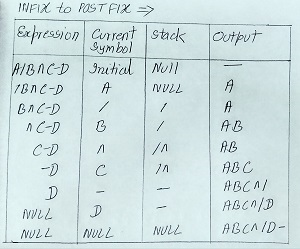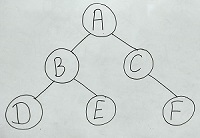Search your topic here

UGC NET June 2012 Paper II solved

UGC NET
Computer Science & Applications, June 2012
PAPER-II
Q1. The postfix expression AB + CD - * can be evaluated using a
(A) Stack
(B) Tree
(C) Queue
Ans: (A)
Explanation: Stack method is used to convert infix to postfix and postfix to infix.
For example, see image.Q2. The post order traversal of a binary tree is DEBFCA. Find out the preorder traversal.
(A) ABFCDE
(C) ABDECF
(D) None of the above
Ans: (C)
Explanation: DFD – Inorder (Left,Root,Right), Preorder (Root,Left,Right), Postorder (Left,Right,Root). So, POSTORDER to BINARY TREE will like this.Than BINARY TREE to PREORDER. Where PREORDER (Root, Left, Right) = ABDECF.
Q3. The branch logic that provides making capabilities in the control unit is known as
(A) Controlled transfer
(B) Conditional transfer
(C) Unconditional transfer
(D) None of the above
Ans: (A)
Q4. The number of colours required to properly colour the vertices of every planar graph is
(A) 2
(B) 3
(C) 4
(D) 5
Ans: (D)
Q5. Networks that use different technologies can be connected by using
(A) Packets
(B) Switches
(C) Bridges
(D) Routers
Ans: (D)
Q6. Both hosts and routers are TCP/IP protocol software. However, routers do not use protocol from all layers. The layer for which protocol software is not needed by a router is
(A) Layer – 5 (Application)
(B) Layer – 1 (Physical)
(C) Layer – 3 (Internet)
(D) Layer – 2 (Network Interface)
Ans: (A)
Q7. In multiuser database if two users wish to update the same record at the same time, they are prevented from doing so by
(A) Jamming
(C) Documentation
(D) Record lock
Ans: (D)
Q8. A binary search tree is a binary tree :
(A) All items in the left subtree are less than root
(B) All items in the right subtree are greater than or equal to the root
(C) Each subtree is itself a binary search tree
(D) All of the above
Ans: (D)
Explanation: A binary tree is- when left node is smaller than root, right node is greater or equal to root, and each subtree is binary tree.
Q9. What deletes the entire file except the file structure ?
(A) ERASE
(B) DELETE
(C) ZAP
(D) PACK
Ans: (C)
Q10. Which command is the fastest among the following ?
(A) COPY TO
(B) COPY STRUCTURE TO
(C) COPY FILE
(D) COPY TO MFILE-DAT DELIMITED
Ans: (B)
Q11. B+ tree are preferred to binary tree in Database because
(A) Disk capacity are greater than memory capacities
(B) Disk access is much slower than memory access
(C) Disk data transfer rates are much less than memory data transfer rate
(D) Disks are more reliable than memory
Ans: (B)
Q12. A Transaction Manager is which of the following ?
(A) Maintains a log of transactions
(B) Maintains before and after database images
(C) Maintains appropriate concurrency control
(D) All of the above
Ans: (D)
Q13. Leaves of which of the following trees are at the same level ?
(A) Binary tree
(B) B-tree
(C) AVL-tree
(D) Expression tree
Ans: (B)
Q14. Which of the following TCP/IP Internet protocol is diskless machine uses to obtain its IP address from a server ?
(A) RAP
(B) RIP
(C) ARP
(D) X.25
Ans: (C)
Q15. Decryption and encryption of data are the responsibility of which of the following layer ?
(A) Physical layer
(B) Data Link layer
(C) Presentation layer
(D) Session layer
Ans: (C)
Q16. In which circuit switching, delivery of data is delayed because data must be stored and retrieved from RAM ?
(A) Space division
(B) Time division
(C) Virtual
(D) Packet
Ans: (B)
Q17. In which Routing Method do all the routers have a common database ?
(A) Distance vector
(D) Dijkestra method
Ans: (B)
Q18. Page Shift Keying (PSK) Method is used to modulate digital signal at 9600 bps using 16 level. Find the line signals and speed (i.e. modulation rate).
(A) 2400 bauds
(B) 1200 bauds
(C) 4800 bauds
(D) 9600 bauds
Ans: (A)
Explanation: To calculate baud rate, modulation have to be divided by the bit rate. See the table below:
 MODULATION BAUD RATE BIT RATE 4-PSK N 2N 8-PSK N 3N 16-PSK N 4N
Here, modulation is 96000 bps which is at level 16, so 96000/4 = 2400 bauds.
Q19. The station to hub distance in which it is 2000 metres.
(A) 100 Base-Tx
(B) 100 Base-Fx
(C) 100 Base-T4
(D) 100 Base-T1
Ans: (B)
Q20. Main aim of software engineering is to produce
(A) Program
(B) Software
(C) Within budget
(D) Software within budget in the given schedule
Ans: (D)
Q21. Key process areas of CMM level 4 are also classified by a process which is
(A) CMM level 2
(B) CMM level 3
(C) CMM level 5
(D) All of the above
Ans: (C)
Q22. Validation means
(A) Are we building the product right
(B) Are we building the right product
(C) Verification of fields
(D) None of the above
Ans: (B)
Explanation: Validation means are we building the right product. Verification means are we building the product right.
Q23. If a process is under statistical control, then it is
(A) Maintainable
(B) Measurable
(C) Predictable
(D) Verifiable
Ans: (C)
Q24. In a function oriented design, we
(A) Minimize cohesion and maximize coupling
(B) Maximize cohesion and minimize coupling
(C) Maximize cohesion and maximize coupling
(D) Minimize cohesion and minimize coupling
Ans: (B)
Q25. Which of the following metric does not depend on the programming language used ?
(A) Line of code
(B) Function count
(C) Member of token
(D) All of the above
Ans: (B)
Q26. A / B+ tree index is to be built on the name attribute of the relation STUDENT. Assume that all students names are of length 8 bytes, disk block are of size 512 bytes and index pointers are of size 4 bytes. Given this scenario what would be the best choice of the degree (i.e. the number of pointers per node) of the B+ tree?
(A) 16
(B) 42
(C) 43
(D) 44
Ans: (A)
Q27. The Inorder traversal of the tree will yield a sorted listing of elements of tree in
(A) Binary tree
(B) Binary search tree
(C) Heaps
(D) None of the above
Ans: (B)
Q28. Mobile IP provides two basic functions.
(A) Route discovery and registration
(B) Agent discovery and registration
(C) IP binding and registration
(D) None of the above
Ans: (B)
Q29. Pre-emptive scheduling is the strategy of temporarily suspending a gunning process
(A) Before the CPU time slice expires
(B) To allow starving processes to run
(C) When it requests I/O
(D) To avoid collision
Ans: (A)
Q30. In round robin CPU scheduling as time quantum is increased the average turn around time
(A) Increases
(B) Decreases
(C) Remains constant
(D) Varies irregularly
Ans: (D)
Q31. Resources are allocated to the process on non-sharable basis is
(A) Mutual exclusion
(B) Hold and wait
(C) No pre-emption
(D) Circular wait
Ans: (A)
Q32. Cached and interleaved memories are ways of speeding up memory access between CPU’s and slower RAM. Which memory models are best suited (i.e. improves the performance most) for which programs ?
(i) Cached memory is best suited for small loops.
(ii) Interleaved memory is best suited for small loops
(iii) Interleaved memory is best suited for large sequential code.
(iv) Cached memory is best suited for large sequential code.
(A) (i) and (ii) are true.
(B) (i) and (iii) are true.
(C) (iv) and (ii) are true.
(D) (iv) and (iii) are true.
Ans: (B)
Q33. Consider the following page trace :
4,3, 2, 1, 4, 3, 5, 4, 3, 2, 1, 5
Percentage of page fault that would occur if FIFO page replacement algorithm is used with number of frames for the JOB m = 4 will be
(A) 8
(B) 9
(C) 10
(D) 12
Ans: (C)
Explanation: F shows page fault using FIFO  page replacement algorithm..
 4 3 2 1 4 3 5 4 3 2 1 5 4 4 4 4 4 4 5 5 5 5 1 1 3 3 3 3 3 3 4 4 4 4 5 2 2 2 2 2 2 3 3 3 3 1 1 1 1 1 1 2 2 2 F F F F H H F F F F F F
Q34. Check sum used along with each packet computes the sum of the data, where data is treated as a sequence of
(A) Integer
(B) Character
(C) Real numbers
(D) Bits
Ans: (D)
Q35. If an integer needs two bytes of storage, then the maximum value of a signed integer is
(A) 216 – 1
(B) 215 – 1
(C) 216
(D) 215
Ans: (B)
Q36. Which of the following logic families is well suited for high-speed operations ?
(A) TTL
(B) ECL
(C) MOS
(D) CMOS
Ans: (B)
Explanation: TTL (Transistor-Transistor-Logic), made of bipolar transistors. ECL(Emitter Coupled Logic), for extremely high speed. NMOS, PMOS for VLSI large scale integrated circuits. CMOS(Complementary Metal Oxide Semiconductor), made from MOSFETs.
Q37. Interrupts which are initiated by an instruction are
(A) Internal
(B) External
(C) Hardware
(D) Software
Ans: (D)
Q38. printf (“%c”, 100);
(A) Prints 100
(B) Prints ASCII equivalent of 100
(C) Prints garbage
(D) None of the above
Ans: (B)
Q39. For the transmission of the signal, Bluetooth wireless technology uses
(A) Time division multiplexing
(B) Frequency division multiplexing
(C) Time division duplex
(D) Frequency division duplex
Ans: (C)
Q40. Consider the following statements:
I. Recursive languages are closed under complementation.
II. Recursively enumerable languages are closed under union.
III. Recursively enumerable languages are closed under complementation.
Which of the above statements are true ?
(A) I only
(B) I and II
(C) I and III
(D) II and III
Ans: (B)
Q41. What is the routing algorithm used by RIP and IGRP ?
(A) OSPF
(C) Dynamic
(D) Dijkestra vector
Ans: (D)
Q42. Identify the incorrect statement :
(A) The overall strategy drives the E-Commerce data warehousing strategy.
(B) Data warehousing in an E-Commerce environment should be done in a classical manner.
(C) E-Commerce opens up an entirely new world of web server.
(D) E-Commerce security threats can be grouped into three major categories.
Ans: (D)
Q43. Reliability of software is directly dependent on
(A) Quality of the design
(B) Number of errors present
(C) Software engineers experience
(D) User requirement
Ans: (B)
Q44. ______ is not an E-Commerce application.
(A) House banking
(C) Conducting an auction
(D) Evaluating an employee
Ans: (D)
Q45. ______ is a satellite based tracking system that enables the determination of person’s position.
(A) Bluetooth
(B) WAP
(C) Short Message Service
(D) Global Positioning System
Ans: (D)
Q46. A complete microcomputer system consists of
(A) Microprocessor
(B) Memory
(C) Peripheral equipment
(D) All of the above
Ans: (D)
Q47. Where does a computer add and compare data ?
(A) Hard disk
(B) Floppy disk
(C) CPU chip
(D) Memory chip
Ans: (C)
Q48. Pipelining strategy is called implement
(A) Instruction execution
(B) Instruction prefetch
(C) Instruction decoding
(D) Instruction manipulation
Ans: (B)
Q49. Which of the following data structure is linear type ?
(A) Strings
(B) Lists
(C) Queues
(D) All of the above
Ans: (D)
Q50. To represent hierarchical relationship between elements, which data structure is suitable ?
(A) Dequeue
(B) Priority
(C) Tree
(D) All of the above
Ans: (C)